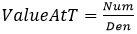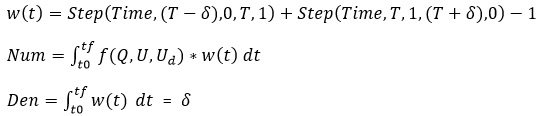# ValueAtTime

Model ElementValueAtTime computes the value of a signal, f(Q,U,Ud)

ValueAtTime

## Description

ValueAtTime computes the value of a signal, f(Q,U,Ud), at a certain time T using:(1)where:## Attribute Summary

Name Property Modifiable by Command? Designable
label Str ()
atTime Double ()
measuredValue Function ()   Yes
delta Double (1.0E-3)
scale Double (1)

## Usage

ValueAtTime (atTime=double, measuredValue=expressionString, delta=double,
optional_attributes)

## Attributes

atTime
Double
Time point at which the output is desired.
atTime is mandatory.
measuredValue
expressionString
A MotionSolve expression defining the function whose value is desired.
measuredValue is mandatory.
Optional attributes
label
String
Specifies the name of the ValueAtTime object.
This attribute is optional. When not specified, MotionSolve creates a label for you.
delta
Double
Interval around atTime to be used in the step function for the calculation of measuredValue.
delta is optional.
(Default: 1.0E-3).
scale
Double
Specifies the scale factor to scale the ValueAtTime value. If not specified, it defaults to 1.0.
This attribute is optional.

## Example:

# Compute the ROLL angle of a marker wrt. ground @ time T=1.2 in degrees

roll = "RTOD*AZ({I})".format(I=m.coupler.cm2.id)

roll12 = ValueAtTime (
label = "Roll at T=1.2",
measuredValue = roll,
delta = 0.02,
atTime = 1.2,
)